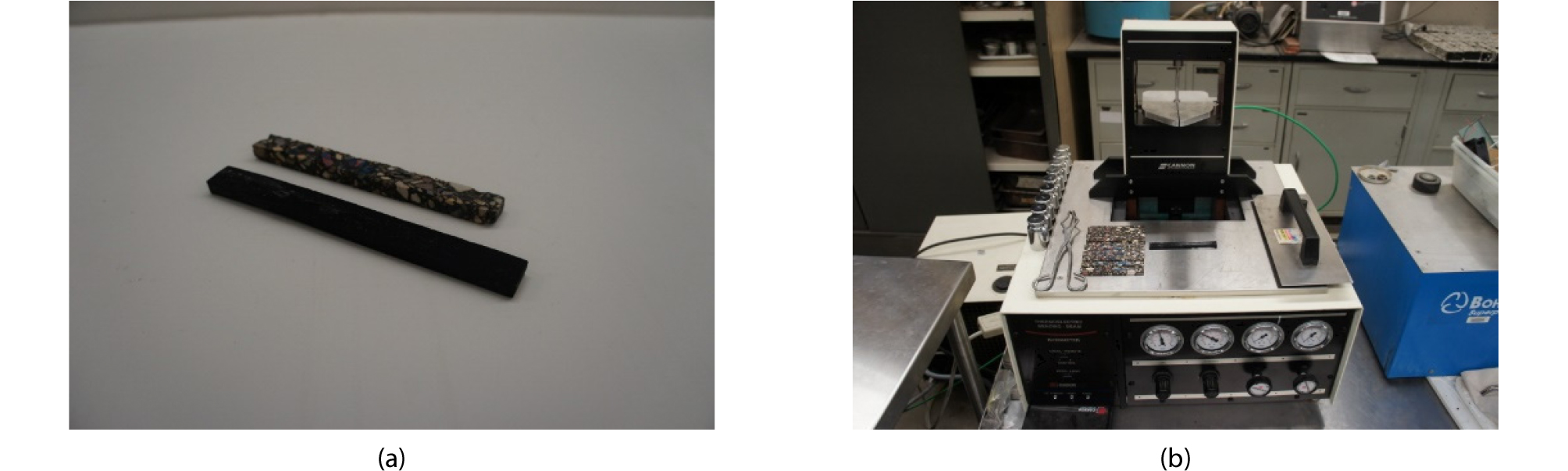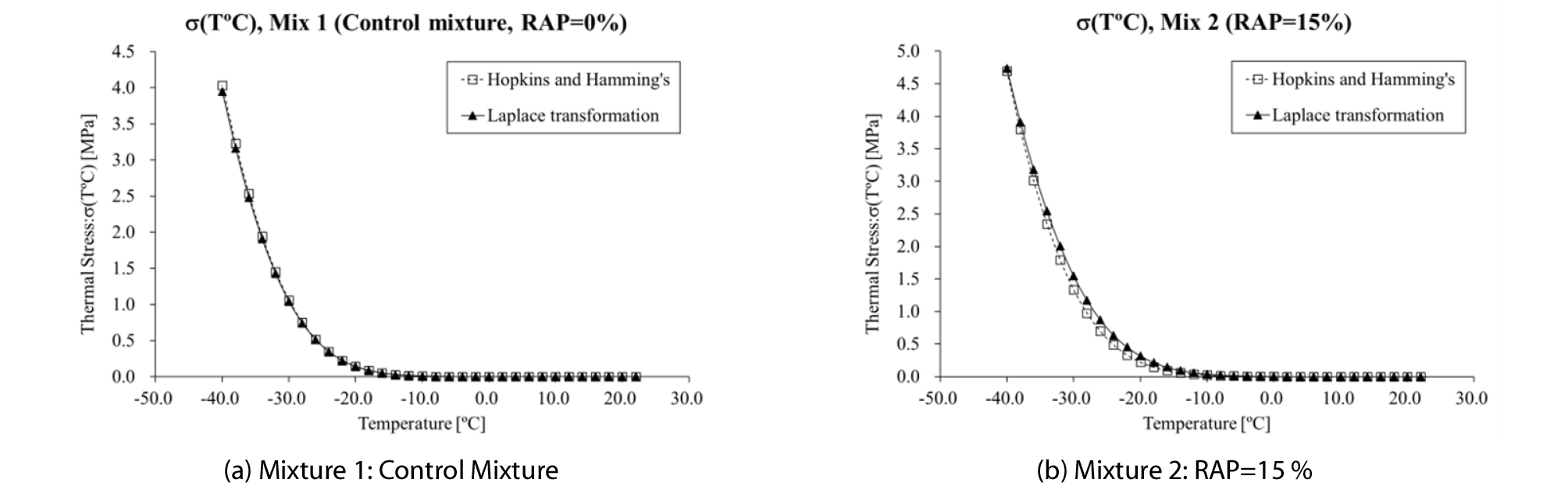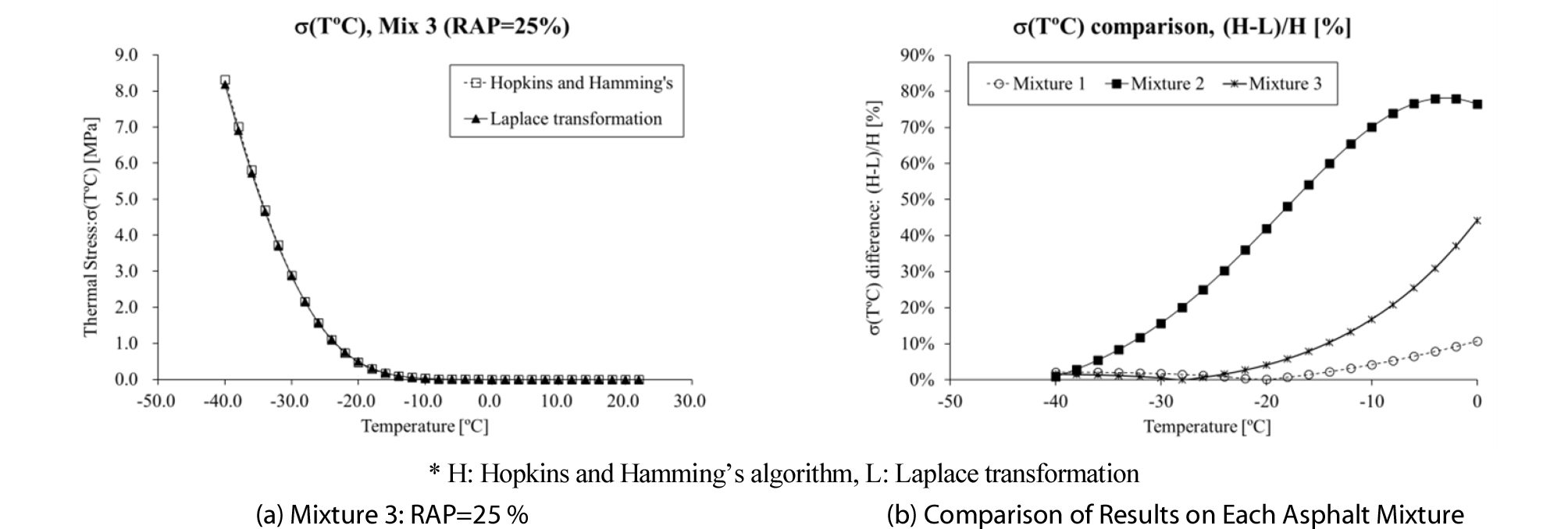# MAIN

• 1. Introduction

• 2. Experimental Works

•   2.1 Asphalt Material Preparations

•   2.2 Bending Beam Rheometer (BBR) Mixture Creep Test

• 3. Two Different Approaches for Computing Thermal Stress of Asphalt Mixture

•   3.1 Traditional Approach using Hopkins & Hamming’s Algorithm (1967) and CAM Model (1999)

•   3.2 Alternative Approach using Laplace Transformation

• 4. Data Analysis (Comparison of Thermal Stress Results)

• 5. Summary and Conclusion

## 1. Introduction

Computing and estimating low temperature performance of asphalt mixture is one of the crucial tasks especially for South Korea, northern U.S. and Canada (Basu, 2002; Velasquez et al., 2009; Moon, 2010; Marasteanu et al., 2009; Moon et. al., 2013; Cannone Falchetto et al., 2018). In many pavement agencies in various countries, the low temperature performance of asphalt pavement layer is measured through the resistance level (and/or value) against longitudinal thermal cracking: the thermal stress and corresponding critical cracking temperature (Moon, 2010; Marasteanu et al., 2009; Moon 2012; Cannone Falchetto et al., 2014a; Cannone Falchetto et al., 2014b; Moon et al., 2014a; Moon et al., 2014b; Cannone Falchetto et al., 2018). Moreover, it is well known that thermal stress and corresponding critical cracking temperature of given asphalt material can be numerically computed (and/or measured) by means of low temperature creep testing (Park and Kim, 1999; Di Benedetto et al., 2009; Moon, 2010; Marasteanu et al., 2009; Moon 2012; Cannone Falchetto et al., 2014a; Cannone Falchetto et al., 2014b; Moon et al., 2014a; Moon et al., 2014b; Cannone Falchetto et al., 2018).

The conventional computation process of thermal stress is consisted of three steps: first, the relaxation modulus: E(t), is generated through the inter-conversion process of the experimental creep compliance data: D(t), by application of Hopkins and Hamming’s algorithm (1967). Secondly, E(t) master curve is generated through various mathematical models and corresponding parameters are computed. Thirdly, thermal stress: 𝜎(T), is numerically computed by solving the E(t) convolution integral (Marasteanu and Anderson, 1999; Basu, 2002; Moon, 2010; Marasteanu et al., 2009; Moon 2012; Cannone Falchetto and Moon, 2015). However, this 𝜎(T) computation approach is relatively complex for many pavement agency employees and researchers to perform. In this paper, an alternative and simple 𝜎(T) computation approach using the Laplace transformation is introduced. As an experimental work, Bending Beam Rheometer (BBR) mixture creep test (Marasteanu et al., 2009; Moon, 2010; Moon, 2012) was performed with 3 different asphalt mixtures. Finally, all the computed 𝜎(T) results by using two different computation approach were compared graphically.

## 2. Experimental Works

2.1 Asphalt Material Preparations

Three different asphalt mixtures were prepared and tested in the asphalt pavement laboratory at Korea Expressway Corporation (KEC). All the mixtures were compacted with a Superpave Gyratory Compactor (SGC) (Marasteanu et al., 2009) to a target air voids content of 7 % (AASHTO M320-10, 2010) with Nominal Maximum Aggregate Size (NMAS) of 12.5 mm. In addition, all the mixtures were designed with a Styrene Butadiene Styrene (SBS) modified asphalt binder containing Performance Grade (PG) 76-34 (AASHTO M320-10, 2010). The overall design binder content was set to 4.2~4.8 %. Two different amounts of Reclaimed Asphalt Pavement (RAP): 15 % and 25 %, were included in the mix design. All the specimens were long term aged based on current AASHTO specification mentioned elsewhere (AASHTO R028-12, 2012). Schematic information of the materials used in this paper is shown in Table 1.

Table 1. Asphalt Mixtures

 Mix ID Binder PG Granite (%) RAP (%) NMAS (mm) VMA (%) Vbe (%) Air (%) Asphalt (%) 1 76-34 (SBS) 100 0 12.5 17.3 10.9 6.4 4.3~4.7 2 85 15 12.5 17.4 10.8 6.6 4.2~4.6 3 75 25 12.5 17.7 11.0 6.7 4.3~4.6

Note: NMAS=Nominal Maximum Aggregate Size, VMA = Voids between Mineral Aggregates, Vbe = Volume of effective asphalt binder, Air = Air void, Asphalt = Portion of asphalt binder, For mixtures 1 to 3, maximum filler content is around 1.4~1.6%, VMA=Vbe+Air

2.2 Bending Beam Rheometer (BBR) Mixture Creep Test

To compute 𝜎(T), Bending Beam Rheometer (BBR) mixture creep experimental works (Marasteanu et al., 2009) were performed on thin asphalt binder mixture beams (length : 102.05 mm width : 12.70.5 mm height : 6.250.5 mm) (see Fig. 1) for 1,000 testing seconds with applying 4,000~6,000 mN of constant load amount.##### Fig. 1.

BBR Binder Mixture Test Specimen (a) and Testing Equipment Setup (b)

Similar to the BBR low temperature creep testing on asphalt binder currently mentioned as testing specification in AASHTO (AASHTO T313-06, 2006), the creep stiffness: S(t) can be computed based on Bernoulli-Euler beam theory in BBR creep mixture test as (Moon, 2010; Moon, 2012):

 $$S(t)=\frac\sigma{\varepsilon(t)}=\frac{P\cdot l^3}{4\cdot b\cdot h^3\cdot\delta(t)}=\frac1{D(t)}$$ (1)

In Eq. (1), D(t), 𝜎, 𝜀(t), P, l, b, h, 𝛿(t) and t mean creep compliance, maximum bending stress, bending strain, applied load, length, width, height, deflection in the mid span and testing time, respectively. To generate relaxation modulus: E(t), master curves and to compute thermal stress: 𝜎(T), the BBR mixture creep tests were performed at two different temperatures: low PG + 10°C and low (PG +10°C)+12°C (i.e. -24: reference temperature and -12°C: higher temperature). Three replicated were used at each testing condition therefore, total six mixtures were used for one set of prepared asphalt mixture in this study.

## 3. Two Different Approaches for Computing Thermal Stress of Asphalt Mixture

3.1 Traditional Approach using Hopkins & Hamming’s Algorithm (1967) and CAM Model (1999)

It is well known that the values of D(t) and E(t), for a viscoelastic material are correlated through the convolution integral as (Findley et al., 1976; Ferry, 1980; Ebrahimi et al., 2014):

 $$\int_0^tE\left(t-\tau\right)\cdot D(t)d\tau=t\;or\;\int_0^tE(t)\cdot D(t-\tau)d\tau=t$$ (2)

Based on Eq. (2), E(t) was generated numerically by applying Hopkins and Hamming’s algorithm (1967) in this paper as following computation steps:

1) Select time interval as:

 $$t_0=0,\;t_1=1,\;t_2=2,\;\cdots\;t_{1,000}=1,000$$ (3)

2) Express the integral form of D(t) and compute f(t) as (see Eq. (4)):

 $$\begin{array}{l}f(t)=\int_0^tD(t)dt\Rightarrow f(t_{n+1})=\\f(t_n)+\frac12\cdot(D(t_{n+1})+D(t_n))\cdot(t_{n+1}-t_n)\end{array}$$ (4)

3) Modify Eq. (2) based on Eq. (4) then express in terms of time: t, as (see Eq. (5)):

 $$\begin{array}{l}t_{n+1}=\int_0^{t_n+1}E(t')\cdot D(t_{n+1}-t')dt'=\\\sum_{i=0}^n\int_{t_i}^{t_i+1}E(t')\cdot D(t_{n+1}-t')dt'\end{array}$$ (5)

4) Approximate each element of the integral in Eq. (5) and rewrite Eq. (6) as (see Eq. (8)):

 $$\begin{array}{l}\int_{t_i}^{t_i+1}E(t')\cdot D(t_{n+1}-t')dt'=\\-E(t_{i+1/2})\cdot\lbrack f(t_{n+1}-t_{t+1})-f(t_{n+1}-t_i)\rbrack\end{array}$$ (6)

where interval time can be expressed as:

 $$t_{i+\frac12}=\frac12\cdot(t_{i=1}+t_i)$$ (7)
 $$\begin{array}{l}t_{n=1}=-\sum_{i=0}^{n-1}E(t_{i+1/2})\cdot\lbrack f(t_{n+1}-t_{i+1})-f(t_{n+1}-t_i)\rbrack\\+E(t_{n+1/2})\cdot f(f_{n+1}-t_n)\end{array}$$ (8)

5) Derive E(t) from Eq. (8) by solving for $E\left({t}_{n+1/2}\right)$

 $$\begin{array}{l}\\E(t_{n+1/2})=\\\begin{array}{l}\frac{t_{n+1}-{\displaystyle\sum_{i=o}^{n-1}}E(t_{i+1/2})\cdot\lbrack f(t_{n+1}-t_i)-f(t_{n+1}-t_{i+1})\rbrack}{f(t_{n+1}-t_n)}=\\\frac{t_{n+1}-{\displaystyle\sum_{i=0}^{n-1}}E(t_{i+1/2})\cdot\lbrack f(t_{i+1})-f(t_i)\rbrack}{f(t_{n+1})-f(t_n)}\end{array}\end{array}$$ (9)
 $$\begin{array}{l}\mathrm{where},\;f(t_0)=0,\;E(_0t)=0,\;E(t_1)=\frac{t_1}{f(t_1)}\\\end{array}$$ (10)

After computation step (5), E(t) master curve was generated based on Christensen, Anderson and Marasteanu (CAM) model (Marasteanu and Anderson, 1999) on BBR experimental data (see Eqs. (11) and (12): Shift factor) as can be seen in Eq. (11):

 $$\begin{array}{l}E(t)=E_g\cdot\left[1+\left(\frac t{t_c}\right)^v\right]^{-\frac wv}\\\end{array}$$ (11)
 $$\begin{array}{l}a_t=10^{C_1+C_2\cdot T}\\\end{array}$$ (12)

In Eq. (11), Eg means glassy modulus (i.e. approximately 30~35 GPa, Moon, 2010; Moon et al., 2013) and tc, v, w, C1, C2 represent fitting parameters. Finally, thermal stress: 𝜎(T), was calculated numerically by solving the following convolution integral:

 $$\begin{array}{l}\sigma(\xi)=\int_{-\infty}^\xi\frac{d\varepsilon(\xi')}{d\xi'}\cdot E(\xi-\xi')d\xi'=\\\int_{-\infty}^t\frac{d(\alpha\cdot\triangle T)}{dt'}\cdot E(\xi(t)-\xi'(t))dt'\\\end{array}$$ (13)
 $$\begin{array}{l}\varepsilon(t)=\alpha\cdot\triangle T\\\end{array}$$ (14)

In Eq. (14), 𝛼 means the coefficient of thermal expansion or contraction; in this study it is assumed as 30.2810-6 (Moon, 2010; Moon et al., 2013). The 𝜎(T) was computed at temperature ranged from 22 to -40°C (temperature interval was 0.5°C) with consideration of 2°C/hour of material cooling rate. Moreover, 24 Gauss points integration (points used for Gauss-Legendre numerical integration) was considered to solve Eq. (12) numerically (Moon, 2010; Moon et al., 2013, Moon et al., 2014b; Moon et al., 2014b). Detailed about the computation process is mentioned elsewhere (Moon, 2010).

3.2 Alternative Approach using Laplace Transformation

As an alternative computation approach for computing 𝜎(T), Laplace transformation was used in this paper. A brief summary of the computation procedure is presented as following steps:

1) Determine the shift factor, aT, and hence, parameters C1 and C2 in Eq. (12) based on the BBR test results (i.e. LogD(t) versus Log(t) curve). Then re-express Eq. (12) as:

 $$\begin{array}{l}a_T=10^{C_1+C_2\cdot T}=10^{C_1+C_2\cdot(T_i-C_o\cdot t)}\\=10^{(C_1+C_2\cdot T_i)-C_2\cdot C_0\cdot t)}=10^{C_3+C_4\cdot t}=A_0\cdot10^{C_4\cdot t}\end{array}$$ (15)

2) Construct the reduce time based master curve of D(𝜉)based on BBR experimental data at two temperature conditions: low PG+10°C and low(PG+10)+12°C, as (see Eq. (16)):

 $$D(\xi)=a\cdot\xi^b+c\cdot\xi^d (16) where: a, b, c and d = Fitting parameters, and$\xi =\frac{t}{{a}_{T}}$= Reduced time (at reference temperature Ti = 22°C). 3) Based on Eq. (13), the relationship between strain and thermal stress can be expressed as: $$\xi_t=\int_0^\xi D(\xi-\xi')\frac{\partial\sigma}{\partial\xi'}d\xi'+\int_0^\xi\alpha(\xi-\xi')\frac{\partial(\triangle T)}{\partial\xi'}d\xi'=0$$(17) By applying the Laplace transformation, Eq. (17) can be rewritten as: $$s\cdot\overline D(s)\cdot\overline\sigma(s)+s\cdot\overline\alpha(s)\cdot\triangle\overline T(s)=0$$(18) $$\sigma(s)=-\frac{\overline\alpha(s)\cdot\triangle\overline T(s)}{\overline D(s)}$$(19) In Eqs. (18) and (19), parameters 𝛼 and T can be expressed as: $$\alpha(t)=\alpha_{0\;}\Rightarrow\;\overline\alpha(s)=\frac{\alpha_0}s$$(20) $$\triangle T=-C_0\cdot t\Rightarrow\triangle\overline T(s)=-\frac{C_0}{s^2}$$(21) 4) Transform (i.e. inverse transformation) Eq. (19) from Laplace domain to reduced time domain (𝜉) using Stehfest algorithm (D.Amore et al., 2018; Stehfest 1970; Villinger H., 1985) then the results of numerically generated 𝜎(𝜉) can then be fitted with the following power function (see Eq. (22)): $$\sigma(\xi)=a+b\cdot\xi^c$$(22) Finally, thermal stress can be computed by converting the thermal stress function from the reduced time domain, 𝜎(𝜉) (Eq. (22)) to the actual time domain, 𝜎(T), with application of Eq. (23): $$\begin{array}{l}\xi=\int_0^t\frac{dt'}{a_T\lbrack T(t')\rbrack}=\int_0^t\frac{dt'}{A_0\cdot10^{C_4\cdot t'}}=\frac1{A_0}\int_0^t10^{-C_4\cdot t'}dt'\\=\frac1A\cdot\left[-\frac{10^{-C_4t'}}{C_4\ln10}\right]_0^t=\frac1{A_0}\cdot\left[\frac1{C_4\ln10}\cdot(1-10^{-C_4t})\right]\\=\frac1{A_0\cdot C_4\cdot\ln10}\cdot\lbrack1-10^{-C_4t}\rbrack=A_1\cdot\lbrack1-10^{-C_4t}\rbrack\end{array}$$(23) $$A_0=10^{C_3}=10^{C_1+C_2\cdot T_i}\;\mathrm{and}\;C_4=-C_2\cdot C_0 (24)

Based on Eqs. (20), (21), (22) and (23), thermal stress was computed at temperature ranged from 22 to -40°C with 0.5°C of temperature interval similar to the previous section. Then the computed results of 𝜎(T) from section 3.1 and 3.2 were visually compared.

## 4. Data Analysis (Comparison of Thermal Stress Results)

From Eqs. (2) to (23), values of 𝜎(T) were computed with using two different computation approaches: traditional Hopkins and Hamming’s algorithm and Laplace transformation. All the results are presented in Table 2 and Figs. 2 and 3.

Table 2. Comparison Results of Computed Thermal Stress [MPa] (Averaged Results)

 Contents Temperature 0°C -2°C -6°C -8°C -10°C -16°C -26°C -36°C -40°C M1(H) 0.0002 0.0005 0.0021 0.0043 0.0083 0.0500 0.5192 2.5339 4.0348 M1(L) 0.0003 0.0004 0.0023 0.0045 0.0086 0.0508 0.5128 2.4807 3.9485 M1(D) 10.7 % 9.2 % 6.5 % 5.3 % 4.2 % 1.5 % 1.2 % 2.1 % 2.1 % M2(H) 0.0016 0.0028 0.0081 0.0137 0.0226 0.0943 0.6984 3.0163 4.6955 M2(L) 0.0029 0.0050 0.0144 0.0237 0.0385 0.1453 0.8727 3.1793 4.7344 M2(D) 76.5% 77.9% 76.5% 73.9% 70.1% 54.1% 25.0% 5.4% 0.8% M3(H) 0.0004 0.0010 0.0055 0.0122 0.0257 0.1720 1.5658 5.8042 8.3136 M3(L) 0.0005 0.0013 0.0070 0.0148 0.0300 0.1856 1.5767 5.7243 8.1811 M3(D) 44.2 % 37.1 % 25.5 % 20.8 % 16.8 % 7.9 % 0.7 % 1.4 % 1.6 %

Note: M1(H): Mixture 1, Hopkins and Hamming's algorithm, M1(L): Mixture 1, Laplace transformation
M1(D): Mixture 1, Differences = ABS[(M(H)-M(L))/M(H)], %##### Fig. 2.

Thermal Stress Comparison Results##### Fig. 3.

Thermal Stress Comparison Results

From the results in Table 2 and Figs. 2 to 3, it can be found that dramatically higher 𝜎(T°C) results were computed with addition of RAP (e.g. 15 % and 25 %). This data generation trend can support the previous findings that addition of RAP provides stiffer characteristics at low temperature compared to the conventional asphalt mixture.

It also needs to mentioned that Laplace transformation provided reasonable and reliable 𝜎(T°C) computation results compared to the conventional 𝜎(T°C) computation method: using Hopkins & Hamming’s algorithm and CAM model (1999). Below the 0°C degree the 𝜎(T°C) result differences between two computation approaches decreased significantly down to 0.8~2.1 % (see Fig. 3). In addition, almost identical 𝜎(T°C) computation results were observed visually between Laplace transformation and Hopkins & Hamming’s algorithm in all different types of asphalt mixture. Based on the findings it can be said the one-step Laplace transformation approach can be a successful alternative for computing low temperature cracking resistant performance of given asphalt materials. In addition, more experimental works and mathematical parametric studies are needed to further narrow the computation results between two different approaches.

## 5. Summary and Conclusion

In this paper, two different computation approaches: Hopkins & Hamming’s algorithm and Laplace transformation, for obtaining thermal stress of three different asphalt mixtures were investigated. As an experimental work, newly developed and modified BBR mixture creep test was performed with various types of asphalt mixture including different portion of RAP. Based on the graphical and numerical comparison some crucial findings, results and limitations can be derived.

(1)It can be said that applying one-step Laplace transformation approach, can successfully be an alternative method for computing thermal stress compared to the conventional and sophisticated computation approach. Based on this approach, it is expected that more university and pavement agency employees are able to compute thermal stress results of asphalt material in more convenient manner than before.

(2)However, only three limited asphalt mixtures and BBR mixture creep testing were considered in this study; therefore, additional extensive experimental work with wider range of asphalt materials and various testing approaches (i.e. IDT creep, IDT strength test) is highly recommended to further verify the findings acquired in this study.

## Acknowledgements

The author would like to gratefully acknowledge the Korea Expressway Corporation Pavement Research Division (KECPRD) for technical support during the experimental work.

본 논문은 2018 CONVENTION 논문을 수정·보완하여 작성되었습니다.

## References

1

American Association of State Highway and Transportation Officials (AASHTO) (2006). Determining the flexural creep stiffness of asphalt binder using the Bending Beam Rheometer (BBR), AASHTO: Specification T313-06, Washington D.C.

2

American Association of State Highway and Transportation Officials (AASHTO) (2010). Standard specification for performance-graded asphalt binder, AASHTO: Specification M320-10, Washington D.C.

3

American Association of State Highway and Transportation Officials (AASHTO) (2012). Standard practice for accelerated aging of asphalt binder using a Pressurized Aging Vessel (PAV), AASHTO: Specification R028-12, Washington D.C..

4

Amore, D. L., Mele, V. and Campagna, R. (2018). "Quality assurance of Gaver΄s formula for multi-precision laplace transform inversion in real case." Inverse Problems in Science and Engineering, Vol. 26, No. 4, pp. 553-580.

10.1080/17415977.2017.1322963
5

Basu, A. (2002). An evaluation of the time-temperature equivalence factor and of the physical hardening effects on low-temperature asphalt binder specifications, M.S. thesis, University of Minnesota, U.S..

6

Cannone, F. A. and Moon, K. H. (2015). "Comparisons of analytical and approximate interconversion methods for thermal stress computation." Canadian Journal of Civil Engineering (CJCE), Vol. 42, No. 10, pp. 705-719.

10.1139/cjce-2014-0558
7

Cannone, F. A., Marasteanu, M. O., Balmurugan, S. and Negulescu, I. I. (2014a). "Investigation of asphalt mixture strength at low temperatures with the bending beam rheometer." Road Materials and Pavement Design (RMPD), Vol. 15, No. 1, pp. 28-44.

8

Cannone, F. A., Le, J.-L., Turos, M. and Marasteanu, M. O. (2014b). "Indirect determination of size effect on strength of asphalt mixtures at low temperatures." Materials and Structures, Vol. 47, pp. 157-169.

10.1617/s11527-013-0052-2
9

Cannone, F. A., Moon, K. H., Wang, D., Riccardi, C. and Wistuba, M. P. (2018). "Comparison of low-temperature fracture and strength properties of asphalt mixture obtained from IDT and SCB under different testing configurations." Road Materials and Pavement Design (RMPD), Vol. 19, No. 3, pp. 591-604.

10.1080/14680629.2018.1418722
10

Di Benedetto, H., Sauzéat, C. and Sohm, J. (2009). "Stiffness of bituminous mixtures using ultrasonic waves propagation." Road Materials and Pavement Design (RMPD), Vol. 10, No. 4, pp. 789-814.

10.1080/14680629.2009.9690227
11

Ebrahimi, M. G., Saleh, M. and Gonzalez, M. A. M. (2014). "Interconversion between viscoelastic functions using the Tikhonov regularization method and its comparison with approximate techniques." Road Material and Pavement Design (RMPD), Vol. 15, No. 4, pp. 820-840.

10.1080/14680629.2014.924428
12

Ferry, J. D. (1980). Viscoelastic properties of polymers, 3rd Edition, Wiley, New York.

13

Findley, W. N., Lai, J. S. and Onaran, K. (1976). Creep and relaxation of nonlinear viscoelastic materials, Dover Publications, New York.

PMC492568
14

Hopkins, I. L. and Hamming, R. W. (1967). "On creep and relaxation." Journal of Applied Physics, Vol. 28, No. 8, pp. 906-909.

10.1063/1.1722885
15

Marasteanu, M. O. and Anderson, D. A. (1999). "Improved model for bitumen rheological characterization." Presented at: European Workshop on Performance-Related Properties for Bituminous Binders, Belgium.

16

Marasteanu, M. O., Velasquez, R. A., Cannone Falchetto, A. and Zofka, A. (2009). Development of a simple test to determine the low temperature creep compliance of asphalt mixtures, IDEA Program Final Report, NCHRP-133.

17

Moon, K. H. (2010). Comparison of thermal stresses calculated from asphalt binder and asphalt mixture creep compliance data, M.S. thesis, University of Minnesota, U.S..

18

Moon, K. H. (2012). Investigation of asphalt binder and asphalt mixture low temperature properties using analogical models, Ph.D. thesis, University of Minnesota, U.S..

19

Moon, K. H., Cannone Falchetto, A. and Yeom, W. S. (2014a). "Low-temperature performance of asphalt mixtures under static and oscillatory loading." Arabian Journal for Science and Engineering (AJSE), Vol. 39, No. 11, pp. 7,577-7,590.

20

Moon, K. H., Cannone Falchetto, A., Park, J. Y., Jeong, J. H. (2014b). "Development of high performance asphalt mastic using fine taconite filler." KSCE J. Civ. Eng., KSCE, Vol. 18, No. 6, pp. 1679-1687.

21

Moon, K. H., Marasteanu M. O. and Turos, M. (2013). "Comparison of thermal stresses calculated from asphalt binder and asphalt mixture creep tests." American Society for Civil Engineers (ASCE): Journal of Materials in Civil Engineering, Vol. 25, No. 8, pp. 1059-1067.

10.1061/(ASCE)MT.1943-5533.0000651
22

Park, S. W. and Kim, Y. R. (1999). "Interconversion between relaxation modulus and creep compliance for viscoelastic solids." American Society for Civil Engineers (ASCE): Journal of materials in Civil Engineering, Vol. 11, No. 1, pp. 76-82.

10.1061/(ASCE)0899-1561(1999)11:1(76)
23

Stehfest, H. (1970). "Algorithm 368: Numerical inversion of Laplace transform." Communication of the Association for Computing Machinery (ACM), Vol. 13, No. 1, pp. 47-49.

10.1145/361953.361969
24

Velasquez, R., Turos, M., Moon, K. H., Zanko, L. and Marasteanu, M. (2009). "Using recycled taconite as alternative aggregate in asphalt pavements." Construction and Building Materials, Vol. 23, No. 9, pp. 3070-3078.

10.1016/j.conbuildmat.2009.04.003
25

Villinger, H. (1985). "Solving cylindrical geothermal problems using Gaver-Stehfest inverse Laplace transform." Geophysics, Vol. 50, No. 10, pp. 1581-1587.

10.1190/1.1441848